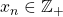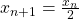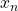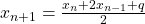# Cycles of higher-order Collatz sequences

John L. Simons
Notes on Number Theory and Discrete Mathematics
Print ISSN 1310–5132, Online ISSN 2367–8275
Volume 28, 2022, Number 1, Pages 48—63
DOI: 10.7546/nntdm.2022.28.1.48-63

## Details

### Authors and affiliations

John L. SimonsUniversity of Groningen
PO Box 800, 9700 AV Groningen, The Netherlands

### Abstract

Consider a sequence of numbersdefined byifis even, andifis odd. A-cycle is a periodic sequence with one transition from odd to even numbers. We prove theoretical and computational results for the existence of-cycles, and discuss a generalization to more complex cycles.

### Keywords

• Collatz problem
• Higher order difference equation
• Linear form in logarithms

• 11B83
• 11J86

### References

1. Baker, A. (1968). Linear forms in the logarithms of algebraic numbers IV. Mathematika, 15, 204–216.
2. Brox, B. (2000). Collatz cycles with few descents. Acta Arithmetica, 92, 181–88.
3. Davison, J. L. (1976). Some comments on an Iteration problem. Proceedings of the 6th Manitoba Conference on Numerical Mathematics (Univ. Manitoba, Winnipeg, Manitoba, 155–159, Congressus Numerantium XVII, Utilitas Math., Winnipeg, MN, 1977.
4. Dujella, A., & Petho, A. (1998). Generalization of a theorem of Baker and Davenport. The Quarterly Journal of Mathematics, 49(3), 291–306.
5. Evertse, J. H. (2007). Linear Forms in Logarithms I: Complex and p-adic. Available online at: http://www.math.leidenuniv.nl/~evertse/linearforms.pdf.
6. Hardy, G. H., & Wright, E. M. (1981). An Introduction to the Theory of Numbers, 5th ed., Oxford University Press.
7. Lagarias, J. C. (2011). The 3x + 1 Problem: An Annotated Bibliography. For latest results see: http://arxiv.org/abs/math.NT/0309224.
8. Laurent, M., Mignotte, M., & Nesterenko, Y. (1995). Formes lineaires en deux logarithmes et determinants d’interpolation. Journal of Number Theory, 55, 285–321.
9. Luca, F. (2005). On the non-trivial cycles in Collatz’s problem. SUT Journal of Mathematics, 41(1), 31–41.
10. Matveev, E. M. (1998). An explicit lower bound for a homogeneous rational linear form in logarithms of algebraic numbers, Part I. Izvestia: Mathematics, 62(4), 723–772.
11. Matveev, E. M. (2000). An explicit lower bound for a homogeneous rational linear form in logarithms of algebraic numbers, Part II. Izvestia: Mathematics, 64(6), 125–180.
12. Mignotte, M. (2008). A kit on linear forms in three logarithms. Available online at: http://irma.math.unistra.fr/~bugeaud/travaux/kit.pdf.
13. Rhin, G. (1987). Approximants de Pade et mesures effectives d’irrationalite. Progress in Mathematics, 71, 155–164.
14. Simons, J. L., & de Weger, B. M. M. (2005). Theoretical and computational bounds for m-cycles of the 3n + 1 problem. Acta Arithmetica, 117.1, 51–70. For latest results see: http://www.win.tue.nl/~bdeweger/research.html.
15. Steiner, R. P. (1978). A Theorem on the Syracuse Problem. Proceeding of 7th Manitoba Conference on Numerical Mathematics 1977, Winnipeg, 553–559.